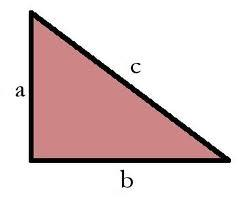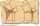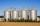# Right triangle Alef

The obvod of a right triangle is 84 cm, and the hypotenuse is 37 cm long. Determine the lengths of the legs.

shorter leg:  12 cm
longer leg:  35 cm

### Step-by-step explanation:

Try calculation via our triangle calculator.Did you find an error or inaccuracy? Feel free to write us. Thank you!Tips to related online calculators
Looking for help with calculating roots of a quadratic equation?
Do you have a linear equation or system of equations and looking for its solution? Or do you have a quadratic equation?

#### You need to know the following knowledge to solve this word math problem:

We encourage you to watch this tutorial video on this math problem:

## Related math problems and questions:

• Right triangleLegs of the right triangle are in the ratio a:b = 2:8. The hypotenuse has a length of 87 cm. Calculate the perimeter and area of the triangle.
• RTriangle 17The hypotenuse of a right triangle is 17 cm. If you decrease both two legs by 3 cm you will reduce the hypotenuse by 4 cm. Determine the length of this legs.
• Right angled triangleThe hypotenuse of a right triangle is 17 cm long. When we decrease the length of legs by 3 cm, then decrease its hypotenuse by 4 cm. Find the size of its legs.
• Right triangle eq2Hypotenuse of a right triangle is 9 cm longer than one leg and 8 cm longer than the second leg. Determine the circumference and area of a triangle.
• Perimeter and legsDetermine the perimeter of a right triangle if the length of one leg is 75% length of the second leg and its content area is 24 cm2.
• Right triangle - ratioThe lengths of the legs of the right triangle ABC are in ratio b = 2: 3. The hypotenuse is 10 cm long. Calculate the lengths of the legs of that triangle.
• Perimeter of RTFind the circumference of the rectangular triangle if the sum of its legs is 22.5 cm and its area is 62.5 cm2.
• Rectangular triangleThe lengths of the rectangular triangle sides with a longer leg of 12 cm form an arithmetic sequence. What is the area of the triangle?
• Euclid3Calculate height and sides of the right triangle, if one leg is a = 81 cm and section of hypotenuse adjacent to the second leg cb = 39 cm.
• RectangleThe perimeter of the rectangle is 22 cm and content area 30 cm2. Determine its dimensions, if the length of the sides of the rectangle in centimeters is expressed by integers.
• Right triangle ABCCalculate the perimeter and area of a right triangle ABC, if you know the length of legs 4 cm 5.5 cm and 6.8 cm is hypotenuse.
• The sidesThe sides of a right triangle form an arithmetic sequence. The hypotenuse is 24 cm long. Determine the remaining sides of the triangle.
• Triangle SASCalculate the triangle area and perimeter, if the two sides are 51 cm and 110 cm long and angle them clamped is 130 °.
• TilesFrom how many tiles 20 cm by 30 cm, we can build a square of maximum dimensions if we have maximum 881 tiles.
• SiloOuter perimeter of silo is 32 m. Concrete wall is 35 cm thick. What is diameter and area of inside floor of silo?
• Area of RTIn the right triangle has orthogonal projections of legs to the hypotenuse lengths 15 cm and 9 cm. Determine the area of ​​this triangle.
• Chickens and rabbitsIn the yard were chickens and rabbits. Together they had 18 heads and 56 legs. How many chickens and how many rabbits were in the yard?